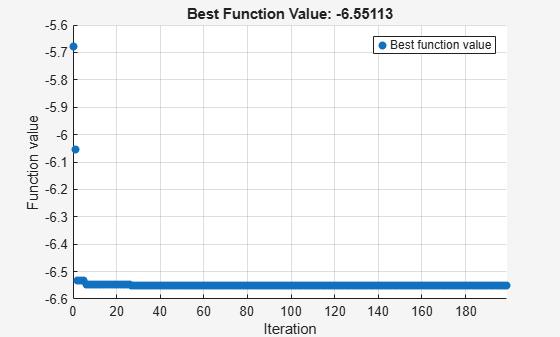# Specify Starting Points and Values for `surrogateopt`, Problem-Based

For some solvers, you can pass the objective and constraint function values, if any, to `solve` in the `x0` argument. This can save time in the solver. Pass a vector of `OptimizationValues` objects. Create this vector using the `optimvalues` function.

The solvers that can use the objective function values are:

• `ga`

• `gamultiobj`

• `paretosearch`

• `surrogateopt`

The solvers that can use nonlinear constraint function values are:

• `paretosearch`

• `surrogateopt`

For example, minimize the `peaks` function using `surrogateopt`, starting with values from a grid of initial points. Create a grid from -10 to 10 in the `x` variable, and `–5/2` to `5/2` in the `y` variable with spacing 1/2. Compute the objective function values at the initial points.

```x = optimvar("x",LowerBound=-10,UpperBound=10); y = optimvar("y",LowerBound=-5/2,UpperBound=5/2); prob = optimproblem("Objective",peaks(x,y)); xval = -10:10; yval = (-5:5)/2; [x0x,x0y] = meshgrid(xval,yval); peaksvals = peaks(x0x,x0y);```

Pass the values in the `x0` argument by using `optimvalues`. This saves time for `solve`, as `solve` does not need to compute the values. Pass the values as row vectors.

```x0 = optimvalues(prob,'x',x0x(:)','y',x0y(:)',... "Objective",peaksvals(:)');```

Solve the problem using `surrogateopt` with the initial values.

`[sol,fval,eflag,output] = solve(prob,x0,Solver="surrogateopt")`
```Solving problem using surrogateopt. ``````surrogateopt stopped because it exceeded the function evaluation limit set by 'options.MaxFunctionEvaluations'. ```
```sol = struct with fields: x: 0.2283 y: -1.6256 ```
```fval = -6.5511 ```
```eflag = SolverLimitExceeded ```
```output = struct with fields: elapsedtime: 45.5839 funccount: 200 constrviolation: 0 ineq: [1x1 struct] rngstate: [1x1 struct] message: 'surrogateopt stopped because it exceeded the function evaluation limit set by ...' solver: 'surrogateopt' ```